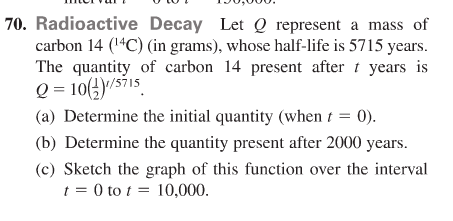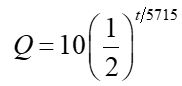# 70. Radioactive Decay Let Q represent a mass ofcarbon 14 (1"C) (in grams), whose half-life is 5715 years.The quantity of carbon 14 present after t years isQ = 10(4)/s71s(a) Determine the initial quantity (when t = 0).(b) Determine the quantity present after 2000 years.(c) Sketch the graph of this function over the intervalt = 0 to t = 10,000.

Question
12 views

only part (c.)  the graphing problemhelp_outlineImage Transcriptionclose70. Radioactive Decay Let Q represent a mass of carbon 14 (1"C) (in grams), whose half-life is 5715 years. The quantity of carbon 14 present after t years is Q = 10(4)/s71s (a) Determine the initial quantity (when t = 0). (b) Determine the quantity present after 2000 years. (c) Sketch the graph of this function over the interval t = 0 to t = 10,000. fullscreen
check_circle

Step 1

Consider the given function...

### Want to see the full answer?

See Solution

#### Want to see this answer and more?

Solutions are written by subject experts who are available 24/7. Questions are typically answered within 1 hour.*

See Solution
*Response times may vary by subject and question.
Tagged in